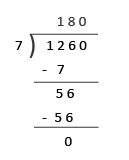## Multiplication and Division

Practice Unlimited Questions

#### 1. Find the product of 1653 and 4. (Multiplying by a 1-digit Number)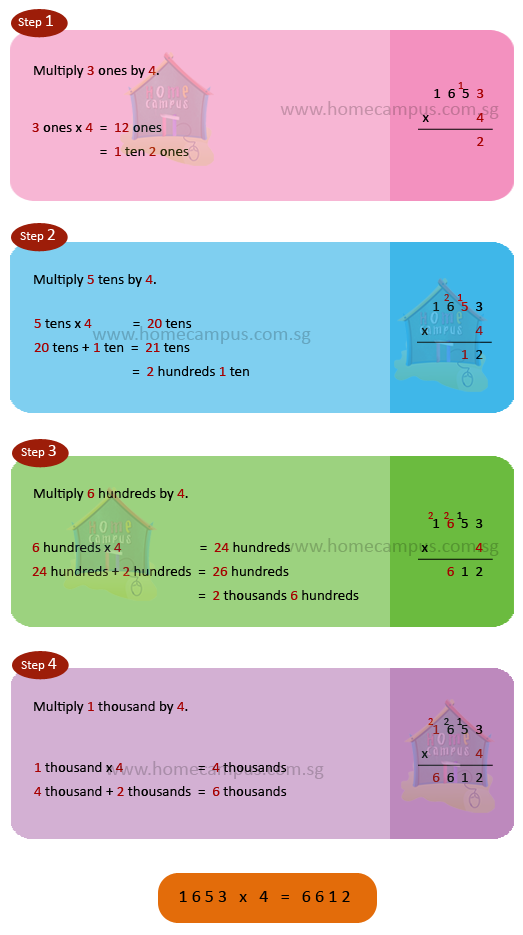#### 2. Multiply 394 by 8.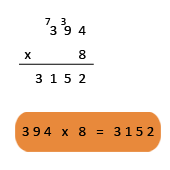#### 3. Multiply 482 by 57. (Multiplying by a 2-digit Number)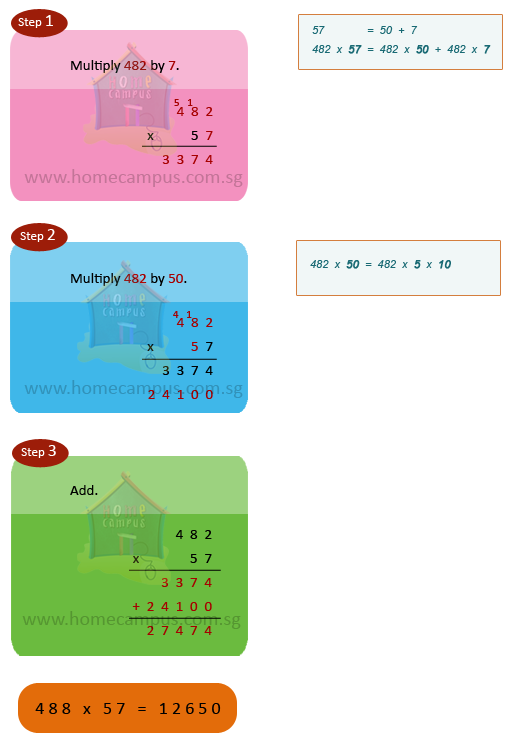#### 4. Find the product of 655 and 28.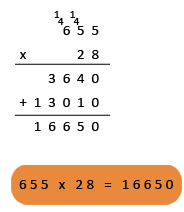#### 5. Divide 8464 ÷ 4. (Dividing by a 1-digit Number)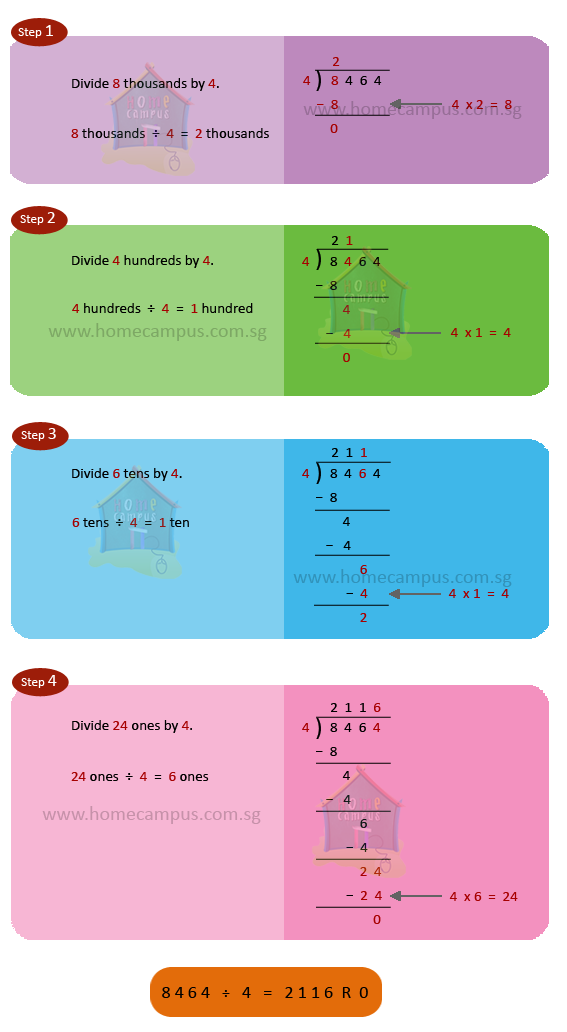#### 6. A baker packed 118 cookies into each tin. a. How many cookies are there in 4 such tins? b. How many cookies are there in 24 such tins?

a.   1 tin   =  118 cookies
4 tins  =  118  ×  4
=   472

There are 472 cookies in 4 tins.b.   1 tin     =  118 cookies
24 tins  =  118  ×  24
=   2832

There are 2832 cookies in 24 tins.#### 7. Mala divided 1260 beads equally to make 7 necklaces. How many beads did she use for each necklace?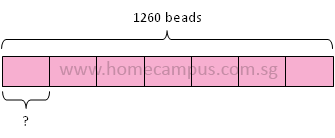1260 ÷ 7  =  180
She used 180 beads for each necklace.